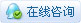# [uPyCraft IDE] 常用的模块 内置函数 3.1.1

#### 1. dir(obj)

``````>>> import sys
>>> dir(sys)
['__name__', 'path', 'argv', 'version', 'version_info', 'implementation', 'platform', 'byteorder', 'maxsize', 'exit', 'stdin', 'stdout', 'stderr', 'modules', 'print_exception']
>>> dir(sys.argv)
['append', 'clear', 'copy', 'count', 'extend', 'index', 'insert', 'pop', 'remove', 'reverse', 'sort']``````

#### 2. encode()

``````>>> str = "DFRobot"
>>> strEn = str.encode()
>>> print(strEn)
b'DFRobot'
>>>
>>> print(str)
F
>>> print(strEn)
70``````

#### 3. find(str[, beg=0, end=len(s)-1])

``````>>> str = "DFRobot"
>>> print( str.find("bo") )
4
>>> print(str.find("Bo"))
-1``````

``````>>> str = "DFRobot"
>>> print(str.find("o", 1))
3
>>> print(str.find("o", 3, 6))
3``````

#### 4. rfind(str[, beg=0, end=len(s)-1])

``````>>> str = "DFRobot"
>>> print(str.rfind("o"))
5
>>> print(str.rfind("B"))
-1``````

``````>>> str = "DFRobot"
>>> print(str.find("o", 1))
3
>>> print(str.rfind("o", 4, 6))
5``````

#### 5. index(str[, beg=0, len=len(s)-1])

``````>>> str = "DFRobot"
>>> print(str.index("o"))
3
>>> print(str.index("B"))
Traceback (most recent call last):
File "<stdin>", line 1, in <module>

``````>>> str = "DFRobot"
>>> print(str.index("o", 2))
3
>>> print(str.index("o", 4, 3))
5``````

#### 6. rindex(str[, beg=0, len=len(s)-1])

``````>>> str = "DFRobot"
>>> print(str.rindex("o"))
5
>>> print(str.rindex("B"))
Traceback (most recent call last):
File "<stdin>", line 1, in <module>

``````>>> str = "DFRobot"
>>> print(str.rindex('o', 2))
5
>>> print(str.rindex('o', 0, 4))
3``````

#### 7. str.join(a)

str：分隔符
a：要连接的列表、元组、字典、字符串等。

``````>>> a = ["hello", "world", "hello", "DFRobot"]
>>> str = ':'
>>> print(str.join(a))
'hello:world:hello:DFRobot'
>>>
>>> a = "hello world"
>>> print(' '.join(a))
'h e l l o   w o r l d'
8. str.split(a[, num])``````

str：分隔符
num：分割次数，

``````>>> str = "www.dfrobot.com.cn"
>>> print(str.split('.'))
['www', 'dfrobot', 'com', 'cn']
>>>
>>> print(str.split('.', 1))
['www', 'dfrobot.com.cn']
>>>
>>> print(str.split('.', 3))
['www', 'dfrobot', 'com', 'cn']
>>>
>>> print(str.split('.', 3))
com``````

#### 9. splitlines([keepends=False])

keepends：是否保留分隔符号
True、False

``````>>> str = "www\rdfrobot\ncom\rnrcn\r\rhello"
>>> print(str.splitlines())  #不保留分隔符
['www', 'dfrobot', 'com', 'nrcn', '', 'hello']
>>>
>>> print(str.splitlines(True))  #保留分隔符
['www\r', 'dfrobot\n', 'com\r', 'nrcn\r', '\r', 'hello']``````

#### 10. rsplit(str, num)

str：分割标志
num：分割次数

``````>>> str = "www.DFRobot.com.cn"
>>> print(str.rsplit('.'))
['www', 'DFRobot', 'com', 'cn']
>>>
>>> print(str.rsplit('.', 1))
['www.DFRobot.com', 'cn']
>>>
>>> print(str.rsplit('.', 3))
['www', 'DFRobot', 'com', 'cn']``````

#### 11. startswith(str[, beg])

str：待检查的字符串
beg：开始检查的位置（字符串下标）。

``````>>> str = "www.dfrobot.com.cn"
>>> print(str.startswith('df'))
False
>>> print(str.startswith('df', 1) )
False
>>> print(str.startswith('df', 4))
True``````

#### 12. endswith(str)

``````>>> str = "www.dfrobot.com.cn"
>>> print(str.endswith('com') )
False
>>> print(str.endswith('cn'))
True``````

#### 13. strip([str])

``````>>> str = "www.dfrobot.com.cn"
>>> print(str.strip()) #去掉空格
www.dfrobot.com.cn
>>> print(str.strip('www') )
.dfrobot.com.cn
>>> print(str.strip('.cn'))
www.dfrobot.com
>>> print(str.strip('com')) #失败，返回原字符串
www.dfrobot.com.cn``````

#### 14. lstrip([str])

``````>>> str = "    www.dfrobot.com.cn"
>>> print(str.lstrip())  #去掉空格
www.dfrobot.com.cn
>>> str = "www.dfrobot.com.cn"
>>> print(str.lstrip('w'))
.dfrobot.com.cn``````

#### 15. rstrip([str])

``````>>> str = "www.dfrobot.com.cn  "  #去掉空格
>>> print(str.rstrip())
www.dfrobot.com.cn
>>>
>>> str = "www.dfrobot.com.cn"
>>> print(str.rstrip('.com.cn'))
www.dfrobot``````

#### 16. format(*args)

format()函数可以接受不限个参数，位置可以不按顺序。

``````>>> print( "{}{}".format("hello", "world") )
helloworld
>>> print( "{1} {0} {1}".format("hello", "world") )
world hello world
>>> print( "1+2={0}, 1*2={1}".format(3, 2) )
1+2=3, 1*2=2``````

format()函数也可以设置参数。

``````>>> str = "web_name:{name}, address:{url}"
>>> site = {"name":"DFRobot", "url":"www.dfrobot.com.cn"}
>>> print(str.format(**site))
>>>
>>> print(str.format(name="DFRobot", url="www.dfrobot.com.cn"))
数字格式化，更多的用法这里不做介绍。

>>> print("{:.2f}".format(3.1415926))
3.14``````

#### 17. replace(old, new[, num])

old：被替换的字符串
new：替换的字符串
num：替换的次数

``````>>> str = "aabbaa"
>>> print(str.replace("aa", "ee"))   #将aa替换成ee
eebbee
>>> print(str.replace("aa", "ee", 1))  #将第一次出现的aa替换成ee
eebbaa``````

#### 18. count(str[, beg, end])

beg（end）：开始（结束）统计的字符串位置。

``````>>> str = "DFRobot"
>>> print(str.count('o'))
2
>>> print(str.count('o', 4))
1
>>> print(str.count('o', 1, 2))
0``````

#### 19. partition(str)

``````>>> str = "Be brave! Break things! Learn and have fun!"
>>> print(str.partition('!'))
('Be brave', '!', ' Break things! Learn and have fun!')``````

#### 20. rpartition(str)

``````>>> str = "DFRobot"
>>> print(str.rpartition('o'))
('DFRob', 'o', 't')``````

#### 21. center(num)

num：填充空格的个数

``````>>> str = "hello"
>>> print(str.center(14), 'flag')
hello      flag``````

#### 22. lower()

``````>>> str = "Be brave! Break things! Learn and have fun!"
>>> print(str.lower())
'be brave! break things! learn and have fun!'``````

#### 23. upper()

``````>>> str = "Be brave! Break things! Learn and have fun!"
>>> print(str.upper())
'BE BRAVE! BREAK THINGS! LEARN AND HAVE FUN!'``````

#### 24. isspace()

``````>>> str = "Be brave! Break things! Learn and have fun!"
>>> print(str.isspace())
False
>>> str = "            "
>>> print(str.isspace())
True``````

#### 25. isalpha()

``````>>> str = "Be brave! Break things! Learn and have fun!"
>>> print(str.isalpha())
False
>>> str = "BebraveBreakthingsLearnandhavefun"
>>> print(str.isalpha())
True``````

#### 26. isdigit()

``````>>> str = "123456"
>>> print(str.isdigit())
True
>>> str = "Be brave! Break things! Learn and have fun!"
>>> print(str.isdigit())
False``````

#### 27. isupper()

``````>>> str = "BE BRAVE! BREAK THINGS! LEARN AND HAVE FUN!"
>>> print(str.isupper())
True
>>> str = "Be brave! Break things! Learn and have fun!"
>>> print(str.isupper())
False``````

#### 28. islower()

``````>>> str = "Be brave! Break things! Learn and have fun!"
>>> print(str.islower())
False
>>> str = "be brave! break things! learn and have fun!"
>>> print(str.islower())
True``````